Technical Information Site of Power Supply Design

• Basic Knowledge
• AC/DC
• Design of a Secondary-Side Synchronous Rectifying Circuit to Improve the Efficiency of an AC/DC Converter
• Synchronous Rectification Circuit Section: Selection of Peripheral Circuit Components－D1, R1, R2 at DRAIN Pin

2020.01.22 AC/DC

# Synchronous Rectification Circuit Section: Selection of Peripheral Circuit Components－D1, R1, R2 at DRAIN Pin

Design of a Secondary-Side Synchronous Rectifying Circuit to Improve the Efficiency of an AC/DC Converter

In the previous article, as the start of design of the synchronous rectification section, the power supply IC used in design was determined. In succession to this, the peripheral circuit components of the selected power supply IC, the BM1R00147F, are selected. In this article, D1, R1, and R2 at the DRAIN pin are selected.

Synchronous Rectification Circuit Section: Selection of Peripheral Circuit Components--D1, R1, R2 at the DRAIN Pin

We begin by considering the circuit section that is examined in this article, in the block diagram of the secondary-side synchronous rectification section shown on the right. We will verify the roles of D1, R1, and R2, inserted between the DRAIN pin of the BM1R00147F and the drain of the MOSFET M2 in the area circled in red, and will set the values for these components.

The voltage at the DRAIN pin of the BM1R00147F controls the gate of the secondary-side MOSFET M2. The DRAIN pin detects the voltage VDS2, but the detected level is as low as several millivolts only, and the slight surge voltage upon switching of the MOSFET M2 is erroneously detected. Hence a means of absorbing surges at the DRAIN pin is necessary.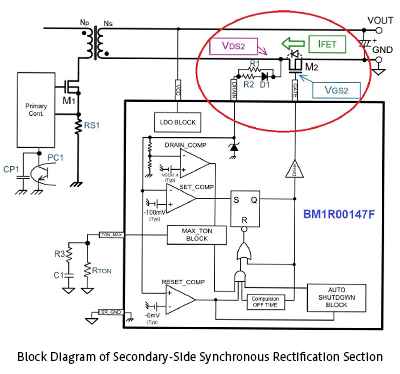Below are a circuit without a surge suppression means at the DRAIN pin, and an example of the resulting waveforms.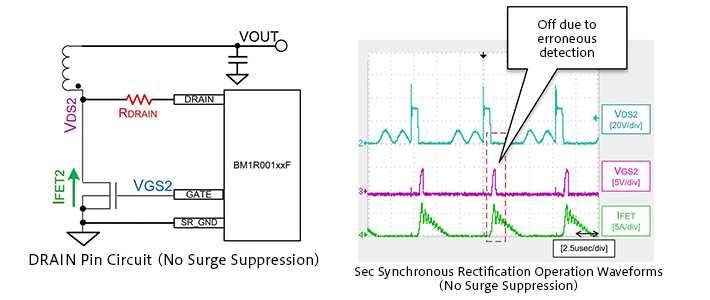In the VDS2 waveform (blue), surges (or spikes) occur at rising edges. As a result, erroneous detection occurs, and VGS2 (purple) turns off for a shorter length of time than is normally required.

Below are shown a circuit with D1, R1, and R2 added at the DRAIN pin as a means of surge suppression, and waveform examples.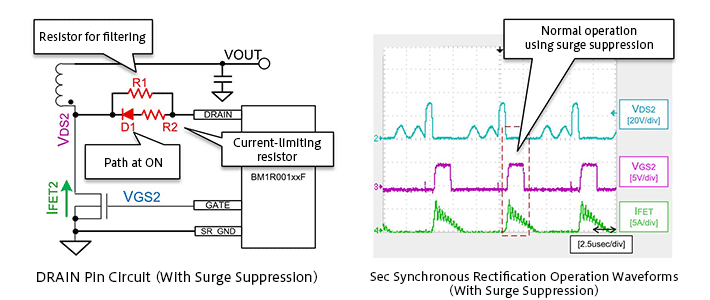By using D1, R1, and R2 to suppress surges, VDS2 is correctly detected, and the VGS2 waveform is also normal.

With this, the reader should understand the reason for inserting D1, R1, and R2, and the effect of the insertion. Next we explain the specific component values set for each of these.

■Diode D1
The diode D1 is a current path when the MOSFET is turned on. In the circuit diagram, a simple diode symbol is shown, but a small-signal Schottky barrier diode (SBD) with a low forward voltage Vf should be selected. Because the DRAIN pin has high impedance, there is no need for D1 to have a rated voltage equal to or higher than VDS2, and a low-voltage component can be chosen. In this design example, a ROHM RB751VM-40 (VR=30 V, IO=30 mA, Vf MAX=0.37 V) is selected.

■R1
R1 is a resistor for VDS2 detection filtering. Approximately 300 Ω to 2 kΩ resistor should be inserted. The resistor should be selected while checking the VDS2 waveform and the VGS2 waveform. In this design case study, a 1 kΩ value is used. A separate more detailed explanation of setting of the R1 value is planned.

■R2
R2 is a current-limiting resistor. At the instant that the current IFET2 begins to flow in the secondary-side MOSFET M2, the MOSFET M2 is in the off state, and so IFET2 flows through the body diode of the secondary-side MOSFET M2. Therefore VDS2 = -Vf_M2 (Vf of the body diode of the MOSFET M2). The DRAIN pin of the IC is at a negative voltage, so that a current Id flows from the IC (See the figure below).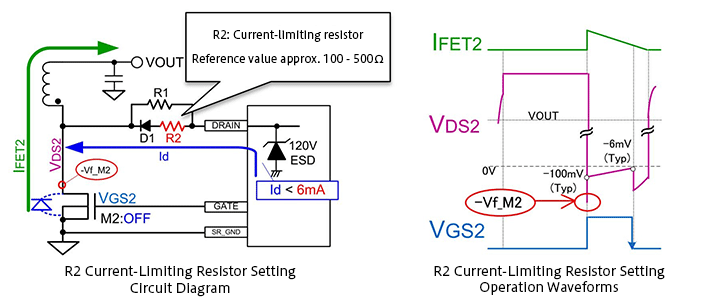To protect the IC, R2 is selected such that the current Id flowing at this time is 6 mA or less. R2 can be calculated using the following formula.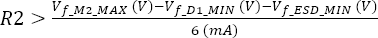When Vf_M2 MAX, which is the maximum value of Vf for the body diode of the MOSFET M2, is 1.2 V, Vf_D1_MIN, which is the minimum value of Vf for D1, is 0.2 V, and Vf_ESD_MIN, which is the minimum value of Vf for the ESD diode within the IC, is 0.4 V, then R2 > 100 Ω.

With a margin included, a 150 Ω resistor is selected.

#### Key Points:

・The voltage at the DRAIN pin of the BM1R00147F controls the gate of the secondary-side MOSFET M2.

・The DRAIN pin detection level is as low as several millivolts, thus the slight surge voltage upon switching of the MOSFET M2 is erroneously detected.

・As a countermeasure, a resistor and diode are added to absorb surges at the DRAIN pin.